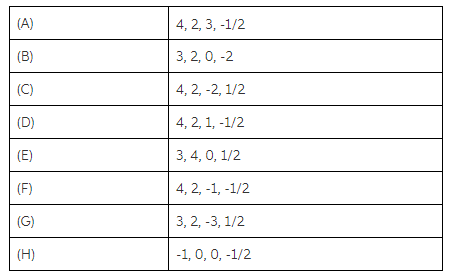# Problem: Part AWhat is the only possible value of ml for an electron in an s orbital?Express your answer numerically.Part BWhat are the possible values of m l for an electron in a d orbital?Express your answer numerically with sequential values separated by commas.Part CWhich of the following set of quantum numbers (ordered n, l, m l, ms) are possible for an electron in an atom?

🤓 Based on our data, we think this question is relevant for Professor Campbell's class at FGCU.

###### Problem Details

Part A

What is the only possible value of ml for an electron in an s orbital?

Part B

What are the possible values of m l for an electron in a d orbital?

Part C

Which of the following set of quantum numbers (ordered n, l, lms) are possible for an electron in an atom?